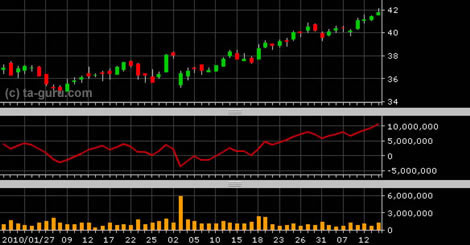Chart Pro online
User name (email):

 Recently used

 Forex

 Indices

# On Balance Volume (OBV)

On balance volume (OBV) indicator is a measure of positive and negative movement of trading volume. The value of the indicator is calculated as follows:

• If the current closing price is higher than previous: OBV (i) = OBV (i-1) + VOLUME (i)
• If the current closing price is less than previous: OBV (i) = OBV (i-1) - VOLUME (i)
• If they are the same: OBV (i) = OBV (i-1)On balance volume (OBV)

Interpretation

The indicator value is not as important as important is direction of its movement. Increased trading volume indicates that a lot of money is invested in the security so increase of price is expected. If growing value of the indicator is seen off with increasing price of security, upward trend is confirmed. However, decline of indicator's value while price is increasing, indicates the possible trend reversal.

Example